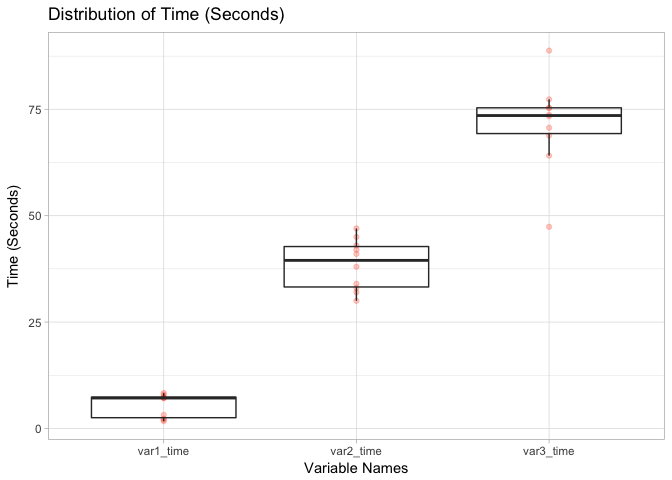# Quick Guide to Create Frequency Table from Wide Table Using R

``````library(dplyr)
library(reshape2)
library(ggplot2)
``````

This post presents a way to display the distribution of multiple variables (with the same comparable unit) side by side and compare their distributions. Normally the data will come in a wide table form in the dataframe, making it impossible to directly use group labels to compare the distributions.

Let’s start by creating an example dataframe:

``````# generate a dataframe with 10 observations and 4 variables
user_id <- seq(1, 10)
var1_time <- runif(10, 1, 10)
var2_time <- sample(seq(30, 50), 10)
var3_time <- rnorm(10, 70, 9)
df <- data.frame(user_id, var1_time, var2_time, var3_time)
glimpse(df)
``````
``````## Observations: 10
## Variables: 4
## \$ user_id   <int> 1, 2, 3, 4, 5, 6, 7, 8, 9, 10
## \$ var1_time <dbl> 2.054537, 8.347375, 7.089574, 3.198027, 1.708012, 7....
## \$ var2_time <int> 38, 41, 33, 34, 32, 43, 30, 45, 47, 42
## \$ var3_time <dbl> 88.80084, 68.84027, 70.66759, 75.27730, 77.33516, 73...
``````

This is what we’ll usually have in our dataframes. The variables are represented horizontally as columns. However, for data visualization purposes, we will align the values of `var1_time`, `var2_time`, and `var3_time` as if they are one variable with different group levels; only in this way can we generate a side by side distribution comparison. Here, we need to turn this `df` into a long table format. In this long format, we will change the dataframe so that it only contains three variables: `user_id`, `var_label`, `time_in_seconds`. The R package `reshape2` is ideal for this task, as shown below:

``````melt(df,
# ID variables, variables not to split
id.vars = c('user_id'),
# Source columns to split
measure.vars = c('var1_time','var2_time','var3_time'),
# Name of the group label variable
variable.name = 'var_name',
# Name of the integrated value
value.name = 'time_in_seconds') -> df_long
glimpse(df_long)
``````
``````## Observations: 30
## Variables: 3
## \$ user_id         <int> 1, 2, 3, 4, 5, 6, 7, 8, 9, 10, 1, 2, 3, 4, 5, ...
## \$ var_name        <fctr> var1_time, var1_time, var1_time, var1_time, v...
## \$ time_in_seconds <dbl> 2.054537, 8.347375, 7.089574, 3.198027, 1.7080...
``````

We can see that `df_long` follows what we desires: it kept the variables we don’t want to split (i.e. `user_id`) but “melted” other variables into one unified measure (i.e. `time_in_seconds`) with “group labels” (i.e. `var_name`). Now We can create a visual to compare the distributions of these three variables side by side, as shown below:

``````g1 <- ggplot(df_long) +
geom_point(aes(x=var_name, y=time_in_seconds), alpha=0.3, color="tomato") +
geom_boxplot(aes(x=var_name, y=time_in_seconds), alpha=0) +
ggtitle("Distribution of Time (Seconds)") + xlab("Variable Names") +
ylab("Time (Seconds)") + theme_light()
g1
``````Tags:

Categories:

Updated: